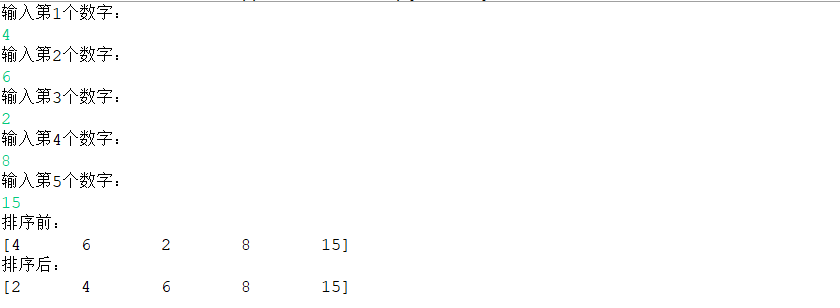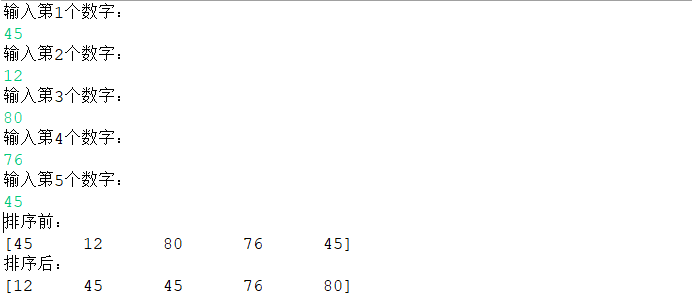• C++实现的简单的冒泡排序排序，简单易懂，运行正常，无bug.
•代码
• 主要介绍了C语言排序算法之冒泡排序实现方法,结合具体实例形式分析了C语言实现的基本冒泡排序实现方法及增设flag标志位的改进型算法,需要的朋友可以参考下
• Java利用选择排序和冒泡排序实现对键盘录入的数据排序
Java利用选择排序和冒泡排序实现对键盘录入的数据排序
选择排序

选择排序是遍历元素找到最大（最小）的元素，放到第一个位置然后到剩余的元素里找到第二大（小）的元素放到第二个，因此类推，就可以得到一个排好序的数组；

使用索引为0的元素依次和后面索引对应的元素进行比较，将较大（小）的值放到前面，第一次比较完毕最大（小）值就在索引为0的位置；
第一次比较是从0索引开始，一直与后面的进行比较，直到比较到最后一个索引；
第二次是从1索引开始，遇到小的互换位置，然后继续比较；
直到最后一个索引；

需求：键盘录入一个数组（5个不同的整数）通过选择排序将数组进行排序并打印

代码实现：
public class ChooseTest {
public static void main(String[] args) {
Scanner sc = new Scanner(System.in);
int[] arr = new int;
for(int i = 0; i <5; i++){
System.out.println("输入第"+(i+1)+"个数字：");
arr[i] = sc.nextInt();
}

System.out.println("排序前：");
printArray(arr);

chooseShot(arr);
System.out.println("排序后：");
printArray(arr);

}
//选择排序
private static void chooseShot(int[] arr) {
for(int i = 0; i < arr.length-1; i++){
for(int j = i +1; j < arr.length; j++){
if(arr[j] < arr[i]){
int temp = arr[i];
arr[i] = arr[j];
arr[j] = temp;
}
}
}
}
//遍历数组
private static void printArray(int[] arr) {
System.out.print("[");
for(int i = 0; i < arr.length; i++){
if(i == arr.length-1){
System.out.println(arr[i] + "]");
}else{
System.out.print(arr[i] + "\t");
}
}
}
}冒泡排序

冒泡排序比较的是相邻的元素，如果第一个数比第二个数大（小），就交换两个元素，没个相邻元素都这么比，直到比较最后一对，此时最后的数应该是最大（小）的；对所有元素重复此操作，就可以得出一个递增（递减）的数组；

0索引和1索引比较，如果0索引大于1索引的值，交换位置否则位置不变
1索引和2索引比较，方法类似

需求：键盘录入一个数组（5个不同的整数）通过冒泡排序将数组进行排序并打印

代码实现：
public class BubbleTest {
public static void main(String[] args) {
Scanner sc = new Scanner(System.in);
int[] arr = new int;
for(int i = 0; i < 5; i++){
System.out.println("输入第"+(i+1)+"个数字：");
arr[i] = sc.nextInt();
}

System.out.println("排序前：");
printArray(arr);

BubbleShot(arr);
System.out.println("排序后：");
printArray(arr);

}
//冒泡排序
private static void BubbleShot(int[] arr) {
for(int i = 0; i < arr.length-1; i++){
for(int j = 0; j < arr.length-1-i; j++){
if(arr[j] > arr[j+1]){
int temp = arr[j];
arr[j] = arr[j+1];
arr[j+1] = temp;
}
}
}
}
//遍历数组
private static void printArray(int[] arr) {
System.out.print("[");
for(int i = 0; i < arr.length; i++){
if(i == arr.length-1){
System.out.println(arr[i] + "]");
}else{
System.out.print(arr[i] + "\t");
}
}
}
}展开全文数据结构 排序算法 java 选择排序
• 主要介绍了shell中的数组操作小结和冒泡排序实现脚本分享,需要的朋友可以参考下
• 初学LabelView写的冒泡排序。 随机产生数组元素，并进行冒泡排序
• 主要介绍了java 数据结构 冒泡排序的相关资料，并附实例代码，有需要的小伙伴可以参考下
• ## C#冒泡排序实现

千次阅读 2018-08-10 17:45:52
冒泡排序：前后两个两两比较，根据需求（从小到大，从大到小）比较  时间复杂度：O（n²） 稳定性：稳定 空间复杂度 O（1） using System; using System.Collections.Generic; using System.Linq; using System....
冒泡排序：前后两个两两比较，根据需求（从小到大，从大到小）比较  时间复杂度：O（n²） 稳定性：稳定  空间复杂度 O（1）
namespace 冒泡排序1 {     class Program     {         static void Main(string[] args)         {             int[] x = new int { 1, 6, 8, 2, 4 };             for (int i = 0; i < x.Length-1; i++)             {                 for (int j = 0; j < x.Length-i-1; j++)                 {                     if (x[j]>x[j+1])    //从小到大排序                     {                         int temp = x[j];                         x[j] = x[j + 1];                         x[j + 1] = temp;                     }                 }             }             for (int i = 0; i < x.Length; i++)             {                 Console.WriteLine("{0}", x[i]);             }             Console.ReadKey();         }     } }  
展开全文• 封装一个函数，利用冒泡排序实现，实现对整形数组的升序排序。 #include<iostream> using namespace std; void print_arr(int* arr, int len); void mp(int* arr, int len); /*冒泡排序函数*/ void mp(int ...
封装一个函数，利用冒泡排序实现，实现对整形数组的升序排序。
#include<iostream>
using namespace std;
void print_arr(int* arr, int len);
void mp(int* arr, int len);

/*冒泡排序函数*/
void mp(int * arr,int len)
{
for (int i = 0; i < len - 1; i++)
{
for (int j = 0; j < len - 1 - i; j++)
{
/*如果j>j+1的值，交换数据*/
if (arr[j] > arr[j + 1])
{
int temp = arr[j];
arr[j] = arr[j + 1];
arr[j + 1] = temp;
}
}
}
}

/*输出函数*/
void print_arr(int* arr, int len)
{
for (int i = 0; i < len; i++)
{
cout << arr[i] << endl;
}
}

int main()
{
/* 1.创建一个数组 */
int arr = { 1,8,5,60,9,11,13,84,16,89,10};
int len = sizeof(arr) / sizeof(arr);
/* 1.创建一个函数，实现冒泡排序 */
mp(arr, len);
/* 1.打印排序后的数组 */
print_arr(arr, len);
return 0;
}



展开全文• * 冒泡排序排序数组从小到大输出 * @author 12136 * */ public class 冒泡排序 { public static void main(String[] args) { int[] arr = {1,3,2,7,5}; int temp; //根据角标进行比较， for(int ...
冒泡
代码示例1：
import java.util.Arrays;

/**
* 冒泡排序排序数组从小到大输出
* @author 12136
*
*/
public class 冒泡排序 {

public static void main(String[] args) {

int[] arr = {1,3,2,7,5};
int temp;
//根据角标进行比较，
for(int i = 0; i<arr.length - 1; i++){
//j是数组的最后一个角标
for (int j = arr.length-1; j > i; j--) {

if (arr[j] < arr[j - 1]) {
//从后往前进行比较，小数往前，一轮之后最小数就在最前面了
temp = arr[j - 1];
arr[j - 1] = arr[j];
arr[j] = temp;
}
}
}

//使用foreach循环输出
for(int x : arr){
System.out.print(x);// 12357
}
System.out.println();
//使用字符串表达形式输出，输出形式更为直观
// [1, 2, 3, 5, 7]
System.out.println(Arrays.toString(arr));
}

}


代码示例2：
public class Test1 {
public static void main(String[] args) {

method1();// 从后往前排：223578
System.out.println();
method2();// 从前往后排：223578

}

private static void method1() {
int arr [] = {5,7,2,2,8,3};

int m;

for (int i = 0; i < arr.length - 1; i++) {
for (int j = arr.length - 1; j > i; j --) {
if (arr[j] < arr[j-1]) {
m = arr[j];
arr[j] = arr[j-1];
arr[j-1] = m;
}
}
}

System.out.print("从后往前排：");
for (int i : arr) {
System.out.print(i);
}

}

private static void method2() {
// TODO Auto-generated method stub
int arr [] = {5,7,2,2,8,3};

int m;

for (int i = 0; i < arr.length - 1; i++) {
for (int j = 1; j <arr.length - i; j ++) {
if (arr[j] < arr[j-1]) {
m = arr[j];
arr[j] = arr[j-1];
arr[j-1] = m;
}
}
}

System.out.print("从前往后排：");
for (int i : arr) {
System.out.print(i);
}

}

}

展开全文java
• * 通过冒泡排序实现从小到大排列 * * 冒泡排序：将数组中第一位与第二位比较，小的数字放在前面，然后再由第二位与第三位作比较，小的数放前面； * 按照这个顺序，得出最大的那个数，并且这个最大的数放在最...
• 操作要求 定义长度为6的整数数组 编写冒泡排序算法，实现数据由大到小的排序
• 自己经验 分享一下，那位需要的 可以看一下 呵呵 帮人就是帮自己嘛
• 冒泡排序： 其基本思想是重复的进行整个数组的排序，一次比较两个元素(两两排序)，如果它们顺序不符合就交换，重复这样直到数列没有再需要交换的数为止(结束条件)。 因为它就好像气泡一样，轻的气泡会往上...
• * 冒泡排序 * 比较次数的公式： N*(N-1)/2 * 原理：相邻元素两两比较，值较大者往后放，第一次比较完毕，最大值出现在了最大索引处。 */ public class BubbleSort { public static void main(String[] args) ...排序
• C#里，输入任何几个学生的成绩，用冒泡排序实现从小到排序。 using System; using System.Collections.Generic; using System.Linq; using System.Text; namespace ConsoleApplication3 {  class Program//随机输入...
• 冒泡排序：是一种从后往前的排序方法，比较相邻的两个数，如果第一个比第二个大就交换它们，依次往下比较，一趟下来就确定了最后的的元素（该数组的最大数）。若该数组有n个元素，需进行n-1趟排序，第i趟比较的次数...
• 理解冒泡排序问题,有详细的步骤 重点: 从某一个值开始,只有当后面的大于此值.交换值, 附代码 public static void main(String[] args) { //1.首先随机生成20个随机数 int[] a=new int; for(int i=0;i<a...
• public static void bubbleSort(int[] arr){ if(arr==null&amp;&amp;arr.length&lt;2){ return; } for (int end = arr.length-1;end&gt;0;end--){ for(int i =...
• 要想做这个题,先要理解什么是冒泡排序. 它重复地走访过要排序的元素列，依次比较两个相邻的元素，如果顺序（如从大到小、首字母从从Z到A）错误就把他们交换过来。走访元素的工作是重复地进行直到没有相邻元素需要...python 列表 字符串
• 2.冒泡排序Java实现 网上冒泡排序算法很多。冒泡排序在工作中，说实话用的比较少。但是，在面试中经常会问到。 package com.evada.de; import org.junit.Test; /** * @Author 阿毅 * Created by Ay on 2016/...jdk 性能优化 Arrays Collection
• ## 冒泡排序实现升序和降序排列

万次阅读 多人点赞 2017-06-06 23:11:49
1.升序排列public static void main(String[] args) { int[] arr = {9,21,34,1,17}; for(int i = 0; i; i++) { for(int j = 0;j;j++) { istring
• data segment table db 'HGFEDCBA' sign db 0 new db 8 dup(0),'\$' how dw 0 data ends code segment assume cs:code,ds:data start: mov ax,data mov ds,ax mov cx,sign-table
• 冒泡排序是较为简单的一种排序方法。 冒泡排序（Bubble Sort，台湾译为：泡沫排序或气泡排序）是一种简单的排序算法。它重复地走访过要排序的数列，一次比较两个元素，如果他们的顺序错误就把他们交换过来。走访数列......